# `sklearn.datasets`.make_circles¶

`sklearn.datasets.``make_circles`(n_samples=100, shuffle=True, noise=None, random_state=None, factor=0.8)[source]

Make a large circle containing a smaller circle in 2d.

A simple toy dataset to visualize clustering and classification algorithms.

Read more in the User Guide.

Parameters: n_samples : int, optional (default=100) The total number of points generated. shuffle : bool, optional (default=True) Whether to shuffle the samples. noise : double or None (default=None) Standard deviation of Gaussian noise added to the data. random_state : int, RandomState instance or None, optional (default=None) If int, random_state is the seed used by the random number generator; If RandomState instance, random_state is the random number generator; If None, the random number generator is the RandomState instance used by np.random. factor : double < 1 (default=.8) Scale factor between inner and outer circle. X : array of shape [n_samples, 2] The generated samples. y : array of shape [n_samples] The integer labels (0 or 1) for class membership of each sample.

## Examples using `sklearn.datasets.make_circles`¶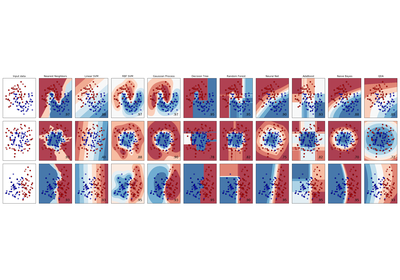Classifier comparison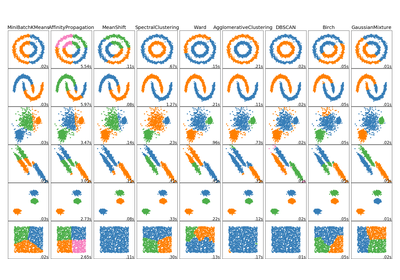Comparing different clustering algorithms on toy datasets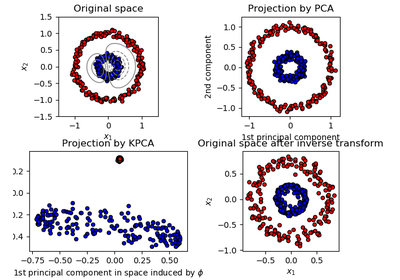Kernel PCA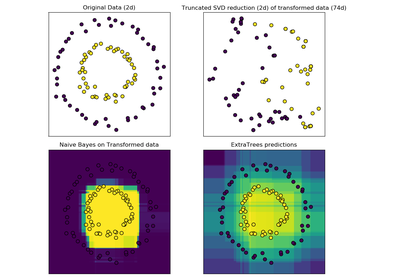Hashing feature transformation using Totally Random Trees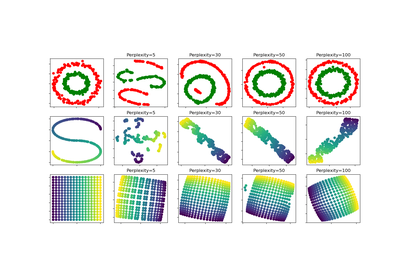t-SNE: The effect of various perplexity values on the shape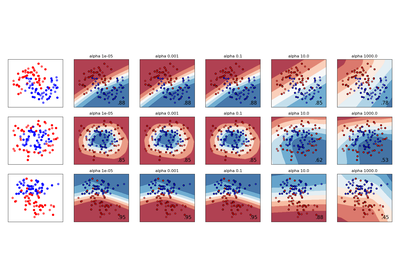Varying regularization in Multi-layer Perceptron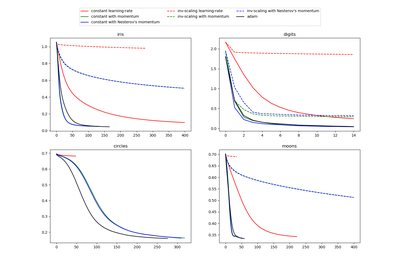Compare Stochastic learning strategies for MLPClassifier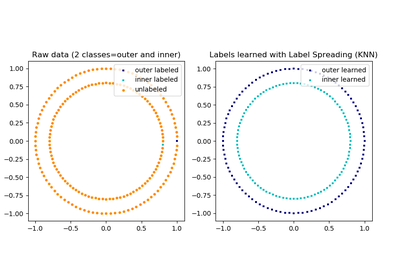Label Propagation learning a complex structure